## Grothendieck's Theorem

Let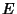andbe paired spaces witha family of absolutely convex bounded sets ofsuch that the sets ofgenerateand, if, then there exists a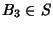such that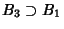and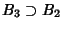. Then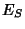is complete Iff algebraic linear functional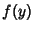ofthat is weakly continuous on everyis expressed asfor some. Whenis not complete, the space of all linear functionals satisfying this condition gives the completionof.

Iyanaga, S. and Kawada, Y. (Eds.). Grothendieck's Theorem.'' §407L in Encyclopedic Dictionary of Mathematics. Cambridge, MA: MIT Press, p. 1274, 1980.